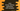# Java Arrays toString method explanation with example## Java Arrays toString method:

Arrays class of Java provides a method called toString to convert the content of an array to a string. This method can be used to convert an array to string easily.

In this post, we will learn how to use toString method with examples.

### Definition of Arrays.toString:

The Arrays.toString method is defined as like below:

``````public static String toString(short[] arr)

public static String toString(byte[] arr)

public static String toString(long[] arr)

public static String toString(int[] arr)

public static String toString(char[] arr)

public static String toString(boolean[] arr)

public static String toString(float[] arr)

public static String toString(double[] arr)

public static String toString(object[] arr)``````

Each of these methods returns the string representation of the contents of the array. The string value will include all elements of the array separated each element by a comma followed by a space and enclosed in a square bracket, []. Each elements are converted to String.

Here, arr is the array to convert to a string and it returns the string representation of the array.

If the array is null, it returns null.

Let me show you how toString works with different types of arrays:

### Example with a short array:

Let’s check it with a short array:

``````import java.util.Arrays;

class Main {
public static void main(String[] args) {
short[] shortArray = {1, 2, 3, 100, 1000};
System.out.println(Arrays.toString(shortArray));
}
}``````

It will print:

``[1, 2, 3, 100, 1000]``

### Example with a byte array:

Let’s use toString with a byte array:

``````import java.util.Arrays;

class Main {
public static void main(String[] args) {
byte[] byteArray = {1, 2, 3, 100, 101};
System.out.println(Arrays.toString(byteArray));
}
}``````

It will print:

``[1, 2, 3, 100, 101]``

### Example with a long array:

Arrays.toString with a long array:

``````import java.util.Arrays;

class Main {
public static void main(String[] args) {
long[] longArray = {10123L, 11222211L, 101011010L};
System.out.println(Arrays.toString(longArray));
}
}``````

It will print:

``[10123, 11222211, 101011010]``

### Example with an integer array:

Example of Arrays.toString with an integer array:

``````import java.util.Arrays;

class Main {
public static void main(String[] args) {
int[] intArray = {1013, 119898, 192829, -999918181};
System.out.println(Arrays.toString(intArray));
}
}``````

It will print:

``[1013, 119898, 192829, -999918181]``

### Example with a character array:

Example of Arrays.toString with a character array:

``````import java.util.Arrays;

class Main {
public static void main(String[] args) {
char[] charArray = {'a', 'e', 'i', 'o', 'u'};
System.out.println(Arrays.toString(charArray));
}
}``````

It will print:

``[a, e, i, o, u]``

### Example with a boolean array:

Example of Arrays.toString with a boolean array:

``````import java.util.Arrays;

class Main {
public static void main(String[] args) {
boolean[] boolArray = {true, false, false, true, true};
System.out.println(Arrays.toString(boolArray));
}
}``````

It will print:

``[true, false, false, true, true]``

### Example with a float array:

Example of Arrays.toString with a float array:

``````import java.util.Arrays;

class Main {
public static void main(String[] args) {
float[] floatArray = {12.23f, 12f, -2.34f};
System.out.println(Arrays.toString(floatArray));
}
}``````

It will print:

``[12.23, 12.0, -2.34]``

### Example with a double array:

Example of Arrays.toString with a double array:

``````import java.util.Arrays;

class Main {
public static void main(String[] args) {
double[] doubleArray = {12.23, -34.45, 23.3455};
System.out.println(Arrays.toString(doubleArray));
}
}``````

It will print:

``[12.23, -34.45, 23.3455]``

### Example with object array:

Let’s try it with an object array:

``````import java.util.Arrays;

class Student {
String name;
int age;

Student(String name, int age) {
this.name = name;
this.age = age;
}
}

class Main {
public static void main(String[] args) {
Student[] students = {new Student("Alex", 12), new Student("Bob", 11)};
System.out.println(Arrays.toString(students));
}
}``````

In this example, Student class is used to hold the name and age of a student. We created one array students with two objects of Student.

We are using Arrays.toString to print the content of this array.

It will print one output as like below:

``[Student@4554617c, Student@74a14482]``

As you can see here, the output is not meaningful. We can override the toString method to make it print the data:

``````import java.util.Arrays;

class Student {
String name;
int age;

Student(String name, int age) {
this.name = name;
this.age = age;
}

@Override
public String toString() {
return "Student{" +
"name='" + name + '\'' +
", age=" + age +
'}';
}
}

class Main {
public static void main(String[] args) {
Student[] students = {new Student("Alex", 12), new Student("Bob", 11)};
System.out.println(Arrays.toString(students));
}
}``````

It will print:

``[Student{name='Alex', age=12}, Student{name='Bob', age=11}]``

You can change the return statement of toString to anything you want.